# Strategic Amortization of Multiple Student Loans¶

## 1. Introduction¶

College is expensive, and students are poor. Thus, students in higher education typically borrow money to pay for school. USA today reported in May, 2017 that 68% of students graduated with debt, which averaged over $30,000 link. The Institute for College Access and Success reports similar numbers specific to Wisconsin link. Fresh graduates are saddled with the responsibility of paying it all back, with interest. However, it's often more complicated than just "paying it back". An auto loan or mortgage is usually taken out as a single loan. The borrower must pay the minimum payment on that loan each month. If the borrower can afford to pay more, then they pay what they can afford each month. There exist a variety of sites and online tools that will calculate the relevant details of the loan payback, or amortization. Given some combination of loan prinicpal, interest rate, monthly payment, or total number of months, there are equations that exist to calculate any other property in the amortization for example. But what if it's not just a single loan? Student loans can only be withdrawn for the coming year. This means that a 4-year program will require a minimum of 4 different loans. Accounting for additional loans for living and other expenses, or if students take out a new loan each semester, or take more than 4 years to graduate, a student could graduate with 8 different loans or more- each with different principals and interest rates. It's a nightmare! So what should a graduate do to best pay off all these loans? If the graduate can only afford the minimum payment, then the choice is already made. But if the graduate is lucky enough to have landed a job out of college and has some excess income that he/she wants to put towards paying off loans more quickly, there are countless strategies available. A Google search for "How to pay off multiple student loans" gives numerous results. The first result suggests one of two methods: 1. Debt avalanche method 2. Debt snowball method The debt snowball method is meant to help gain psychological confidence in paying loans back by focusing on smallest loans first. The debt avalanche method, however, is meant to save the borrower money by focusing on the highest interest loans first. But is it the optimal strategy? There are other considerations when paying back loans. If our graduate's budget is flexible, they are probably trying to decide how much they should pay each month, and what the consequences are for different monthly payments. Optimization can help with this, too. Finally, there are some practical considerations that arose during the exploration of this problem. I will explain these considerations and propse a solution. In this work, I aim to investigate student loan repayment strategies and design a function that will calculate the optimal amortization schedule (schedule of payments) for any given set of loan totals and interest rates. I will also look at some practical considerations and use modeling strategies to answer questions and provide guidance that an indebted graduate could benefit from. ## 2. Mathematical Model¶ Modeling the payback of multiple loans is fairly straightforward with monthly payments on each loan being the decision variables. The main constraints are typical: sum of payments must be less than maximum monthly payment, inital loan balances set to the principals, constraints on subsequent loan balances being subject to interest on a monthly basis. However, there is one quirk of loan payoffs that cannot be modeled as a linear program. Loan servicers typically have a set of constraints on the amount of payments that can be made. The minimum payments are set as a percentage of the current loan balance. A global minimum also exists, meaning that no payments can be made below a certain value. This mean that when loan balances get low enough, the percent minimum payment will be only a few dollars or so. The loan servicer doesn't want to deal with a bunch of \$.50 payments. So, they say that no payments can be made less than \5. But of course, you can't pay more than the balance of the individual loan. The payments that are allowed to be made vs the balance of the loan end up being something like what is plotted below, where the light green overlay are the allowed payments for any given loan balance. The exact numbers are artificially chosen to emphasize the changing modes. In : using PyPlot # loan starting balance maxB = 100 # minimum monthly payment percent # set artificially high for display purposes perc = .2 # global minimum monthly payment glob = 5 x = linspace(0,maxB,100) # plot the balance over time # which is equal to the maximum payment y_balance = x # percent minimum monthly payment y_percent = perc*x # global minimum monthly payment y_global = 0*x + glob # plot the different lines plot(x, y_balance, "k--", linewidth = 2, label="Maximum Payment") hold plot(x, y_percent, "r--", linewidth = 2, label="Percent Minimum") hold plot(x, y_global, "b", linewidth = 2, label="Global Minimum") hold # set limits with inverse x-axis # to illustrate loan payoff xlim([maximum(x),minimum(x)]) ylim([0,maximum(x)]) grid() xlabel("Loan Balance") ylabel("Payment amount") title("Payments allow vs loan balance") # calculate vertices for fill xfill = [maxB,glob/perc,glob,maxB] yfill = [perc*maxB,glob,glob,maxB] fill(xfill, yfill, color= "lightgreen", label="Allowed Payments") legend(loc="upper right",fancybox="true") # extra line for last bit of payments x_final = linspace(0,glob,10) y_final = x_final plot(x_final,y_final, color="lightgreen") ;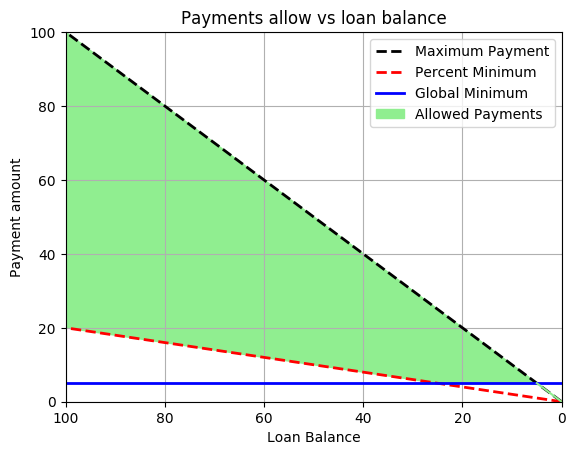As you can see, there are 3 different modes to the minimum monthly payment. 1. Loan balance is high enough that the percent minimum is greater than the global minimum. In this mode, the minimum payment is just the percent minimum. 2. Loan balance is above the global minimum, but the percent minimum is lower than the global minimum. In this mode, the minimum payment is the global minimum. 3. Loan balance is below the global minimum. In this mode, the only payment you can make is to pay off the loan completely. While these different modes may seem trivial, they actually have a significant effect on the optimal amortization of the loans. Without minimum payments being enforced properly, the solver could create a strategy that breaks the loan servicer's constraints in order to find a more optimal strategy. Indeed, without needing to pay a minimum payment on each loan, or paying less than the required minimum payment, the solver finds much better solutions by avoiding low interest rate loans until the end of amortization. In fact, these solutions are infeasible in actual loan payoff and thus misleading. ### 2.A. Model 1¶ The minimum payment allowed can be stated as follows: If A is the greater of 1. the minimum percent payment 2. the minimum global payment then the minimum payment allowed is the lesser of 1. A 2. The balance of the loan The inital model in standard form is then as follows: \begin{aligned} \underset{P \in \mathbb{R^2}}{\text{minimize}}\qquad& \sum_{i,j} P(i,j) \\ \text{subject to:}\qquad& B(1,j) = L(j) \\[.2em] & B(N,j) = 0 \\[.2em] & B(i+1,j) = B(i,j) \cdot (1 + r(j)) - P(i,j) \\[.2em] & P(i,j) \geq \inf\left\{\sup\left\{m \cdot B(i,j),M\right\},B(i,j)\right\}\\[.2em] & P(i,j) \leq B(i,j) \\[.2em] & \sum_j P(i,j) \leq MP \end{aligned} Where •P(i,j)$is a payment made in month$i$on loan$j$•$B(i,j)$is the balance in month$i$of loan$j$•$r(j)$is the interest rate of loan$j$•$L(j)$is the initial principal balance of loan$j$• MP is the maximum payment that the individual can make each month •$m$is the minimum percent allowed by the loan servicer •$M$is the global minimum payment allowed. •$N$is the maximum number of months of the optimization We will model the minimum monthly constraint using a big M constraint, where binary variables are used to decide if the balance of the loan is within the range of the global minimum payment or not. These constraints are specifically defined in code in Section 3.C. around line 58. One final note about the design of the model is that the solver is given a fixed number of months$Nfor which to solve. This number need be greater than or equal to the number of months required to payoff the loans completely, and must be decided in advance. To speed the solving time, this number should be chosen as close to the actual number of required months as possible to avoid excess and unncessary computation. In this work, the number of months for which to solve was set as: $$N = 2*\frac{\sum_j L(j)}{MP}$$ This calculation is not perfect since compound interest is not linear. When the ratio $$\frac{\sum_j L(j)}{MP}$$ is small, then N is calculated much too large. When the ratio is large, N could become lower than the actual number of months required. However, this rough estimate is effective for most realistic cases and thus a more accurate estimate was not needed. ### 2.B. Model 2¶ A second model will be defined. The motivation will be explained in the analysis, but for clarity we will define the model here. Additions in this model include a second term in the objective, and an additional constraint dealing with the introduced variable that is in the objective. \begin{aligned} \underset{P \in \mathbb{R^2}}{\text{minimize}}\qquad& \sum_{i,j} P(i,j) + \lambda \sum_{i,j} \left| D(i,j) \right|\\ \text{subject to:}\qquad& B(1,j) = L(j) \\[.2em] & B(N,j) = 0 \\[.2em] & B(i+1,j) = B(i,j) \cdot (1 + r(j)) - P(i,j) \\[.2em] & P(i,j) \geq \inf\left\{\sup\left\{m \cdot B(i,j),M\right\},B(i,j)\right\}\\[.2em] & P(i,j) \leq B(i,j) \\[.2em] & \sum_j P(i,j) \leq MP\\[.2em] & D(i,j) = P(i,j) - P(i-1,j) \end{aligned} Where •D(i,j)$is the difference between payments made in months$i$and$i-1$•$\lambda$is a parameter set before optimization This model uses an L1 norm on the differences between payments month to month so as to encourage sparsity in the differences. ### 2.C. Assumptions¶ Some assumptions will be made in the solution to this problem. They can be summarized as follows: 1. The graduate's budget remains constant, i.e. the maximum monthly payment does not change for the duration of amortization 2. The loan servicer parameters stay constant. In the real world they could change but their models are opaque so we will use values that were obtained empirically. It's reasonable to assume these values wouldn't have much variation between different servicers. 3. No fees. Usually loan fees are built into the principal and don't need to be considered, so this is a good assumption. ## 3. Solution¶ Various approaches to a solution will be designed and calculated here. A narrative analysis will follow this section that describes the results found. This section can be referred back to for details on the calculations of the results that are shown in section 4. Some of these code cells take several minutes to run. They will be noted as such at the top of the code cell. ### 3.A. Setup of loan details¶ #### Loan Parameters¶ First, the details of the loans that are being paid off need to be provided. Enter the principal values of each loan, the annual interest rates, and the maximum monthly payment that can be afforded. These values are currently set to a real example of a recent college graduate from University of Wisconsin- Madison, with a mix of loan balances and interest rates. This is the example on which the analysis will focus. However, the model is designed to function with any reasonable combination of values. A range of values was tested to ensure differences were only quantitative and there were no significant qualitative differences. Note that the testing was not exhaustive so inputting extreme values may cause errors unrelated to the optimization itself. In : # user-defined parameters # balances of each loan loan_amounts = [3853.15, 339.58, 2081.82, 1230.25, 7625.3, 7084.73, 3635.25] # sum to total principal total_principal = sum(loan_amounts) # corresponding interest rates in annual form int_rates = [4.29, 4.29, 4.29, 4.66, 4.66, 3.86, 6.8] # convert to monthly percentages interest = int_rates./1200 # the monthly payment that can be afforded max_monthly_payment = 350 ;  #### Loan Servicer Parameters¶ If known, edit the values for the minimum payments allowed. These values are dependent on the loan servicer. If not known, use the defaults In : # Percent of balance of loan that must be paid each month min_percent = 0.6 # Global minimum payment- cannot make a payment less # than this amount min_amt = 5 ;  In : # Check for minimum payment error if max_monthly_payment < min_amt*length(loan_amounts) throw(ArgumentError("Monthly payment is too low")) end if max_monthly_payment < sum(min_percent/100*loan_amounts) throw(ArgumentError("Monthly payment is too low")) end  ### 3.B. Loan Payoff Without Optimization¶ First, let's check what happens if a naive approach is used. We will examine the two methods mentioned above: snowball and avalanche. To be precise, the snowball method is defined as paying the minimum required payment on each loan. Then, any monthly leftover payment will be applied to the remaining loan with the smallest balance until all loans are paid off. The avalanche method is defined as paying the minimum required payments on each loan. Then, any monthly leftover payment will be applied to the remaining loan with the highest interest rate until all loans are paid off. Both of these methods will be simulated for the above defined loan parameters to calculate the length of payoff and the total amount paid. In : # Simulate snowball amortization of loans function Snowball(loan_amounts,interest,max_monthly_payment, min_percent, min_amt) # starting loan balances balances = loan_amounts # running balances and payments for plotting running_balances = zeros(1000,length(loan_amounts)) running_payments = zeros(1000,length(loan_amounts)) # dummy variable for use in determining # lowest loan remaining upper = 2*maximum(balances) # counter for amount paid nonopt_total = 0 # counter for number of months num_months = 0 # loop while there is balance left while any(balances .>0) # add interest balances = balances.*(1+interest) # determine which loans are not paid off remaining_loans = balances .> 0 # determine minimum payments minpay = min_percent/100*balances minpay = max.(minpay,min_amt) minpay = min.(minpay,balances) # apply monthly payments balances = balances - minpay running_payments[num_months+1,:] = running_payments[num_months+1,:] + minpay # sum miniumum payments and subtract from monthly payment total_minpay = sum(minpay) amount_left = max_monthly_payment-total_minpay # update total payment amount nonopt_total = nonopt_total + total_minpay while amount_left > 0 && any(balances .>0) # determine which loans are still not paid off remaining_loans = balances .> 0 # determine remaining loan with lowest value minind = indmax(upper*remaining_loans-balances) # pay lowest loan and subtract from amount left low_pay = min(amount_left,balances[minind]) balances[minind] = balances[minind]-low_pay amount_left = amount_left-low_pay nonopt_total = nonopt_total + low_pay running_payments[num_months+1,minind] = running_payments[num_months+1,minind] + low_pay # if the lowest loan was paid off, amount_left # will be positive, and will be passed to next lowest # interest end num_months = num_months + 1 running_balances[num_months,:] = balances end # clean up running balances and payments snowball_balances = vcat(loan_amounts',running_balances[1:num_months,:]) snowball_payments = running_payments[1:num_months,:] snowball_months = num_months snowball_total = nonopt_total return snowball_balances, snowball_payments, snowball_months, snowball_total end ;  In : # Simulate avalanche amortization of loans function Avalanche(loan_amounts,interest,max_monthly_payment, min_percent, min_amt) # starting loan balances balances = loan_amounts # running balances and payments for plotting running_balances = zeros(1000,length(loan_amounts)) running_payments = zeros(1000,length(loan_amounts)) # counter for amount paid nonopt_total = 0 # counter for number of months num_months = 0 # loop while there is balance left while any(balances .>0) # add interest balances = balances.*(1+interest) # determine which loans are not paid off remaining_loans = balances .> 0 # determine minimum payments minpay = min_percent/100*balances minpay = max.(minpay,min_amt) minpay = min.(minpay,balances) # apply monthly payments balances = balances - minpay running_payments[num_months+1,:] = running_payments[num_months+1,:] + minpay # sum miniumum payments and subtract from monthly payment total_minpay = sum(minpay) amount_left = max_monthly_payment-total_minpay # update total payment amount nonopt_total = nonopt_total + total_minpay while amount_left > 0 && any(balances .>0) # determine which loans are still not paid off remaining_loans = balances .> 0 # determine remaining loan with maximum interest rate maxind = indmax(remaining_loans.*interest) # pay highest loan and subtract from amount left high_pay = min(amount_left,balances[maxind]) balances[maxind] = balances[maxind]-high_pay amount_left = amount_left-high_pay nonopt_total = nonopt_total + high_pay running_payments[num_months+1,maxind] = running_payments[num_months+1,maxind] + high_pay # if the highest loan was paid off, amount_left # will be positive, and will be passed to next highest # interest rate loan. end num_months = num_months + 1 running_balances[num_months,:] = balances end # clean up running balances and payments avalanche_balances = vcat(loan_amounts',running_balances[1:num_months,:]) avalanche_payments = running_payments[1:num_months,:] avalanche_months = num_months avalanche_total = nonopt_total return avalanche_balances, avalanche_payments, avalanche_months, avalanche_total end ;  In : snowball_balances, snowball_payments, snowball_months, snowball_total = Snowball(loan_amounts,interest,max_monthly_payment, min_percent, min_amt) ;  In : avalanche_balances, avalanche_payments, avalanche_months, avalanche_total = Avalanche(loan_amounts,interest,max_monthly_payment, min_percent, min_amt) ;  ### 3.C. Loan Payoff With Optimization¶ Now, let's allow the payments to be optimized. The model that was defined in Sec. 2 above is created and optimized to minimize the sum of payments made during the duration of amortization. In : using JuMP, Mosek, NamedArrays function OptimizePayments(loan_amounts,int_rates,max_monthly_payment, min_percent, min_amt) # approximate maximum length of amortization approx_max_months = Int(round(2*sum(loan_amounts)/max_monthly_payment)) months = 1:approx_max_months loans = 1:length(loan_amounts) # convert interest rates to monthly decimals int_rates_m = int_rates/1200 # turn min percent into array decimal min_percent = repmat([min_percent]/100,length(loan_amounts)) # turn global min into array min_amt_array = repmat([min_amt],length(loan_amounts)) # Big Ms for conditional constraints M1val = 1.5*maximum(loan_amounts) M1 = repmat([M1val],length(loan_amounts)) M2 = repmat([2*min_amt],length(loan_amounts)) # Creation of Model m = Model(solver= MosekSolver(LOG=0)) ## Variables # payment[i,j] is payment made towards loan i in month j @variable(m, payment[loans,months] >= 0) # balance[i,j] is balance of loan i at the beginning of month j @variable(m, balance[loans,months] >= 0) # minpay[i,j] is the mininmum payment allowed for loan i # at the beginning of month j @variable(m, minpay[loans,months] >= min_amt) # z is binary variable for enforcing conditional constraint # for minimum payment @variable(m, z[loans,months], Bin) # max payment is a dummy variable that allows us # to find dual of the maximum monthly payment @variable(m, max_payment) @constraint(m, maxpay, max_payment<= max_monthly_payment) # total payment amount @expression(m, total, sum(sum(payment))) ## Constraints # interest enforcement for mm in 2:months[end] @constraint(m, balance[:,mm] .== (1+int_rates_m).*balance[:,mm-1] - payment[:,mm-1]) end # initial principal constraints @constraint(m,init[i in loans], balance[i,1] == loan_amounts[i]) # final payoff constraints @constraint(m, balance[:,months[end]] .== 0) # total monthly payment constraints @constraint(m, pay[j in months], sum(payment[i,j] for i in loans) <= max_payment) # minimum monthly payment constraints # minimum for each loan for mm in months @constraint(m, (min_percent).*balance[:,mm] .<= payment[:,mm]) end # conditional constraint: if Balance >= global minimum, # then Payment >= global mininum using Big-M constraints for mm in months @constraint(m, balance[:,mm] .<= min_amt_array + (M1).*(1-z[:,mm])) @constraint(m, min_amt_array .<= payment[:,mm] + (M2).*z[:,mm]) @constraint(m, balance[:,mm] .<= payment[:,mm] + (M1).*(1-z[:,mm])) end ## Objective # minimize total payments and differences in payments @objective(m, Min, total) # solve status = solve(m) # get values balances = [getvalue(balance[i,j]) for i in loans, j in months] payments = [getvalue(payment[i,j]) for i in loans, j in months] # calculate months to payoff balances = round.(balances,2)' balances[balances.<0.01] = 0 inds = find(balances) subs = ind2sub.([balances], inds) last_month = maximum([ x for x in subs ]) # truncate months for displaying balances = balances[1:last_month+1,:] # create amortization table amortization = NamedArray( balances, (collect(1:last_month+1),collect(loans)), ("Month","Loan")) # format payments for displaying payments = [getvalue(payment[i,j]) for i in loans, j in months] payments = round.(payments,2)' payments[payments.<0.01] = 0 payments = payments[1:last_month,:] amortization_p = NamedArray( payments, (collect(1:last_month),collect(loans)), ("Month","Loan")) return status, getvalue(total), amortization, amortization_p, last_month end ;  This section takes a minute or two to run In : # Run optimizing function status, orig_total, orig_balances, orig_payments,orig_num_months = OptimizePayments(loan_amounts,int_rates, max_monthly_payment, min_percent, min_amt) println(status)  Optimal  ### 3.D. Optimize for perturbed monthly payments¶ This section will calculate the gains or losses that will be achieved by different values of monthly payment This section takes several minutes to run In : # Run amortization over multiple monthly payments N = 11 perturbs = linspace(.5,1.5,N) max_payment_array = perturbs*max_monthly_payment various_opt_totals = zeros(size(max_payment_array)) various_opt_nMonths = zeros(size(max_payment_array)) various_sb_totals = zeros(size(max_payment_array)) various_sb_nMonths = zeros(size(max_payment_array)) various_ava_totals = zeros(size(max_payment_array)) various_ava_nMonths = zeros(size(max_payment_array)) for mm in 1:N curmaxpay = max_payment_array[mm] # Optimized solution _, total, _, _, months = OptimizePayments(loan_amounts,int_rates, curmaxpay, min_percent, min_amt) various_opt_totals[mm] = total various_opt_nMonths[mm] = months # snowball solution _, _, months, total = Snowball(loan_amounts,interest, curmaxpay, min_percent, min_amt) various_sb_totals[mm] = total various_sb_nMonths[mm] = months # avalanche solution _, _, months, total = Avalanche(loan_amounts,interest, curmaxpay, min_percent, min_amt) various_ava_totals[mm] = total various_ava_nMonths[mm] = months end ;  ### 3.E. Solve Trade-off problem with sparse payment changes¶ The motivation for this model design will be described below in the analysis In : using JuMP, Mosek, NamedArrays function SolveTradeoff(loan_amounts,int_rates,max_monthly_payment, λ, min_percent, min_amt) # approximate maximum length of amortization approx_max_months = Int(round(2*sum(loan_amounts)/max_monthly_payment)) months = 1:approx_max_months loans = 1:length(loan_amounts) # convert interest rates to monthly decimals int_rates_m = int_rates/1200 # turn min percent into array decimal min_percent = repmat([min_percent]/100,length(loan_amounts)) # turn global min into array min_amt_array = repmat([min_amt],length(loan_amounts)) # Big Ms for conditional constraints M1val = 1.5*maximum(loan_amounts) M1 = repmat([M1val],length(loan_amounts)) M2 = repmat([2*min_amt],length(loan_amounts)) # Creation of Model m = Model(solver= MosekSolver(LOG=0)) ## Variables # payment[i,j] is payment made towards loan i in month j @variable(m, payment[loans,months] >= 0) # balance[i,j] is balance of loan i at the beginning of month j @variable(m, balance[loans,months] >= 0) # minpay[i,j] is the mininmum payment allowed for loan i # at the beginning of month j @variable(m, minpay[loans,months] >= min_amt) # z is binary variable for enforcing conditional constraint # for minimum payment @variable(m, z[loans,months], Bin) # diffs is the differences from # month to month loan payments # duffs is the dummy variable for absolute value @variable(m, diffs[loans,1:months[end-1]]) @variable(m, duffs[loans,1:months[end-1]]) # total payment amount @expression(m, total, sum(sum(payment))) ## Constraints # interest enforcement for mm in 2:months[end] @constraint(m, balance[:,mm] .== (1+int_rates_m).*balance[:,mm-1] - payment[:,mm-1]) end # initial principal constraints @constraint(m,init[i in loans], balance[i,1] == loan_amounts[i]) # final payoff constraints @constraint(m, balance[:,months[end]] .== 0) # total monthly payment constraints @constraint(m, pay[j in months], sum(payment[i,j] for i in loans) <= max_monthly_payment) # minimum monthly payment constraints # minimum for each loan for mm in months @constraint(m, (min_percent).*balance[:,mm] .<= payment[:,mm]) end # conditional constraint: if Balance >= global minimum, # then Payment >= global mininum using Big-M constraints for mm in months @constraint(m, balance[:,mm] .<= min_amt_array + (M1).*(1-z[:,mm])) @constraint(m, min_amt_array .<= payment[:,mm] + (M2).*z[:,mm]) @constraint(m, balance[:,mm] .<= payment[:,mm] + (M1).*(1-z[:,mm])) end # Smoothness formulation @constraint(m, diffcon[ll in loans, mm in 2:months[end]], diffs[ll,mm-1] == payment[ll,mm]-payment[ll,mm-1]) @constraint(m, diffconL[ll in loans, mm in 1:months[end-1]], diffs[ll,mm] <= duffs[ll,mm]) @constraint(m, diffconG[ll in loans, mm in 1:months[end-1]], diffs[ll,mm] >= -duffs[ll,mm]) @expression(m, duffsum, sum(sum(duffs))) ## Objective # minimize total payments and differences in payments @objective(m, Min, total+λ*duffsum) # solve status = solve(m) # get objective values opt_total = getvalue(total) opt_duffsum = getvalue(duffsum) # get other values balances = [getvalue(balance[i,j]) for i in loans, j in months] payments = [getvalue(payment[i,j]) for i in loans, j in months] # calculate months to payoff balances = round.(balances,2)' balances[balances.<0.01] = 0 inds = find(balances) subs = ind2sub.([balances], inds) last_month = maximum([ x for x in subs ]) # truncate months for displaying balances = balances[1:last_month+1,:] # create amortization table amortization = NamedArray( balances, (collect(1:last_month+1),collect(loans)), ("Month","Loan")) # format payments for displaying payments = [getvalue(payment[i,j]) for i in loans, j in months] payments = round.(payments,2)' payments[payments.<0.01] = 0 payments = payments[1:last_month,:] amortization_p = NamedArray( payments, (collect(1:last_month),collect(loans)), ("Month","Loan")) return status, opt_total, opt_duffsum, amortization, amortization_p, last_month end ;  This section takes quite a while to run In : # Run amortization over multiple lambda N = 11 lams = logspace(-3,1,N) tradeoff_totals = zeros(N) tradeoff_diffsums = zeros(N) for ll in 1:N λ = lams[ll] _, total, diffsum, _, _, _ = SolveTradeoff(loan_amounts,int_rates, max_monthly_payment, λ, min_percent, min_amt) tradeoff_totals[ll] = total tradeoff_diffsums[ll] = diffsum end  This section takes a minute or two to run In : # Run function at a chosen λ λopt = 1e-1 status , totalλopt, _, _, paymentsλopt,num_monthsλopt = SolveTradeoff(loan_amounts,int_rates, max_monthly_payment, λopt, min_percent, min_amt) println(status)  Optimal  ## 4. Results, Analysis and Discussion¶ ### 4.A. Initial Results and Analysis¶ In : println("With the non-optimized snowball strategy:") println(@sprintf("Loan total is \$%.2f",sum(loan_amounts)))
println(@sprintf("Total payoff cost will be \$%.2f", snowball_total)) println(@sprintf("Interest paid will be \$%.2f",snowball_total-sum(loan_amounts)))
println(@sprintf("The loans will be paid off in %d months", snowball_months))
println("")
println("With the non-optimized avalanche strategy:")
println(@sprintf("Loan total is \$%.2f",sum(loan_amounts))) println(@sprintf("Total payoff cost will be \$%.2f", avalanche_total))
println(@sprintf("Interest paid will be \$%.2f",avalanche_total-sum(loan_amounts))) println(@sprintf("The loans will be paid off in %d months", avalanche_months)) println("") println("With the optimized solution:") println(@sprintf("Loan total is \$%.2f",total_principal))
println(@sprintf("Total payoff cost will be \$%.2f", orig_total)) println(@sprintf("Interest paid will be \$%.2f",orig_total-total_principal))
println(@sprintf("The loans will be paid off in %d months", orig_num_months))
println("")
println(@sprintf("Optimizing saves \$%.2f over snowball approach", snowball_total-orig_total)) println(@sprintf("Optimizing saves \$%.2f over avalanche approach", avalanche_total-orig_total))

With the non-optimized snowball strategy:
Loan total is $25850.08 Total payoff cost will be$30357.79
Interest paid will be $4507.71 The loans will be paid off in 87 months With the non-optimized avalanche strategy: Loan total is$25850.08
Total payoff cost will be $30072.25 Interest paid will be$4222.17
The loans will be paid off in 86 months

With the optimized solution:
Loan total is $25850.08 Total payoff cost will be$30071.89
Interest paid will be $4221.81 The loans will be paid off in 86 months Optimizing saves$285.90 over snowball approach
Optimizing saves $0.36 over avalanche approach  There are multiple observations to make on these results. First, clearly the snowball method is the wrong way to go for saving money. While it's understandable to wish to reduce the overwhelming feeling of debt by paying off small loans quickly, both the avalanche method and the optimized approach reduced the total amount paid AND the number of months required to pay all the loans off! ### 4.B. Perturbation Analysis¶ A recent graduate who landed themselves a job is probably budgeting for how much they can afford to pay towards their loans each month. We can provide some guidance by looking at the effects of adjusting the monthly payment on how much the graudate will end up paying over the life of the loans. In : # plot maximum monthly payments curve using PyPlot figure(figsize=(8,4)) plot(max_payment_array, various_opt_totals, "-.o", label="Optimal") plot(max_payment_array, various_sb_totals, "--o", label="Snowball") plot(max_payment_array, various_ava_totals, "--", label="Avalanche") axis([max_payment_array,max_payment_array[end],.95*minimum(various_opt_totals),1.05*maximum(various_opt_totals)]); xlabel("Monthly Payment") ylabel("Total Cost") title("Total Cost vs Monthly Payments Made") grid() legend(loc="upper right", fancybox="true") ;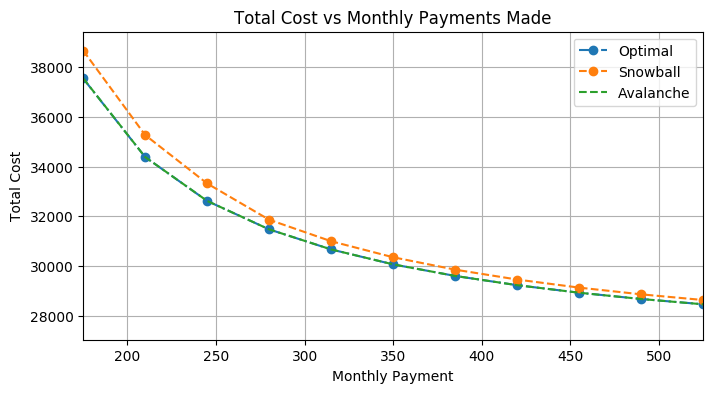As shown in the plot above, there are diminishing returns on paying a larger monthly payment. If the graduate was already planning on paying \$350 per month, pushing that payment to \$400 per month only saves the graduate a few hundred dollars over the life of the loans. However, if the graduate was planning on paying \$200 a month but considering budgeting for \$250, they could end up saving over \$2000! That is a significant amount and could provide the graduate some motivation in finding the extra cash in their budget.

Additionally, we can notice that the differences between the different methods shrink as the monthly payment gets larger.

We can also look at the number of months until payoff- another very important figure to an indebted graduate.

In :
# plot other maximum monthly payments curve
using PyPlot
figure(figsize=(8,4))
plot(max_payment_array, various_opt_nMonths, "--o",label="Optimal")
plot(max_payment_array, various_sb_nMonths, "--o",label="Snowball")
plot(max_payment_array, various_ava_nMonths, "--",label="Avalanche")
axis([max_payment_array,max_payment_array[end],.95*minimum(various_opt_nMonths),1.05*maximum(various_opt_nMonths)]);
xlabel("Monthly Payment")
ylabel("Number of Months to Payoff")
title("Number of Months vs Monthly Payments Made")
grid()
legend(loc="upper right", fancybox="true")
;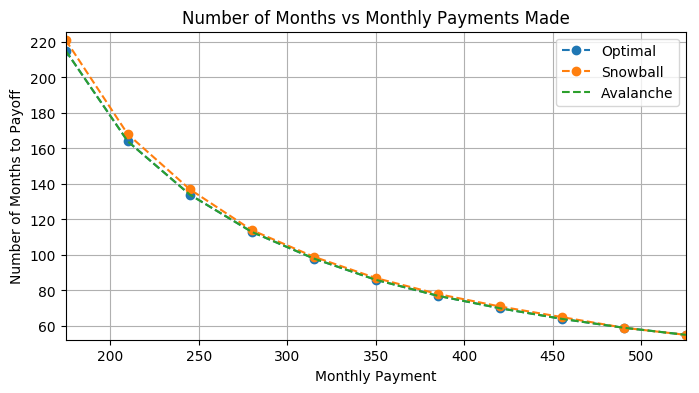The curve is the same shape, which makes sense. Fewer months of payoff is equivalent to smaller total payoff amount since fewer payments are being made.

However, the numbers are perhaps still surprising. The example before considered upping a \$200 monthly payment to \$250. That would result in a faster payoff of almost 50 months, or about 4 years! That is definitely an exciting fact for a graduate to consider when preparing a budget.

Digging deeper into the analysis, it is perhaps quite suprising that a fully optimal approach only beats out a simple strategy like the avalanche approach by a slim margin! (For this example it's only $0.36; it depends on the loan values and maximum monthly payment). Perhaps optimizing isn't helping much, after all. However, there is more that optimizing can do to help an indebted graduate than just with total amount paid. Let's examine the plots of loan balances and payments for each method. The plots will be shown labeled by the annual interest rate of the loan. In : # plot snowball balances using PyPlot figure(figsize=(10,3)) for ll in 1:size(snowball_balances) plot(collect(1:snowball_months+1), snowball_balances[:,ll], "-o",markersize=2,label=int_rates[ll]) end axis([0,snowball_months+1,0,1.05*maximum(snowball_balances)]); xlabel("Months") ylabel("Loan Balance") title("Loan Balances over amortization with Snowball method") legend(loc="upper right", fancybox="true") ; # plot snowball payments using PyPlot figure(figsize=(10,3)) for ll in 1:size(snowball_payments) plot(collect(1:snowball_months), snowball_payments[:,ll], "-o",markersize=2,label=int_rates[ll]) end axis([0,snowball_months+1,0,1.05*maximum(snowball_payments)]); xlabel("Months") ylabel("Payment amount") title("Payments over amortization with Snowball method") legend(loc="upper right", fancybox="true") ; # plot avalanche balances using PyPlot figure(figsize=(10,3)) for ll in 1:size(avalanche_balances) plot(collect(1:avalanche_months+1), avalanche_balances[:,ll], "-o",markersize=2,label=int_rates[ll]) end axis([0,avalanche_months+1,0,1.05*maximum(avalanche_balances)]); xlabel("Months") ylabel("Loan Balance") title("Loan Balances over amortization with Avalanche method") legend(loc="upper right", fancybox="true") ; # plot avalanche payments using PyPlot figure(figsize=(10,3)) for ll in 1:size(avalanche_payments) plot(collect(1:avalanche_months), avalanche_payments[:,ll], "-o",markersize=2,label=int_rates[ll]) end axis([0,avalanche_months+1,0,1.05*maximum(avalanche_payments)]); xlabel("Months") ylabel("Payment amount") title("Payments over amortization with Avalanche method") legend(loc="upper right", fancybox="true") ; # plot optimal balances using PyPlot figure(figsize=(10,3)) for ll in 1:size(orig_balances) plot(collect(1:orig_num_months+1), orig_balances[:,ll], "-o",markersize=2,label=int_rates[ll]) end axis([0,orig_num_months+1,0,1.05*maximum(orig_balances)]); xlabel("Months") ylabel("Loan Balance") title("Loan Balances over amortization with optimization") legend(loc="upper right", fancybox="true") ; # plot optimal payments using PyPlot figure(figsize=(10,3)) for ll in 1:size(orig_payments) plot(collect(1:orig_num_months), orig_payments[:,ll], "-o",markersize=2,label=int_rates[ll]) end axis([0,orig_num_months+1,0,1.05*max_monthly_payment]); xlabel("Months") ylabel("Payment amount") title("Payments over amortization with optimization") legend(loc="upper right", fancybox="true") ;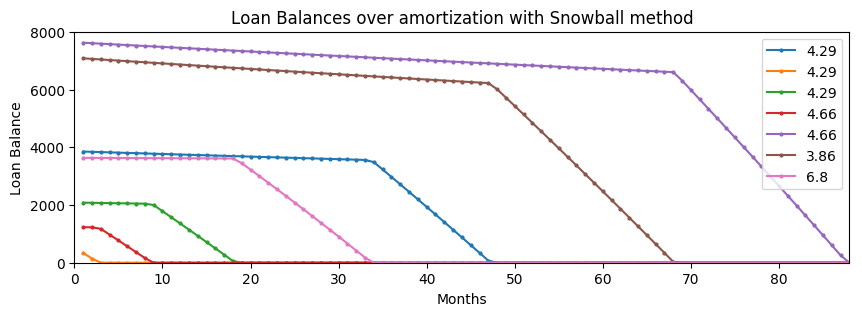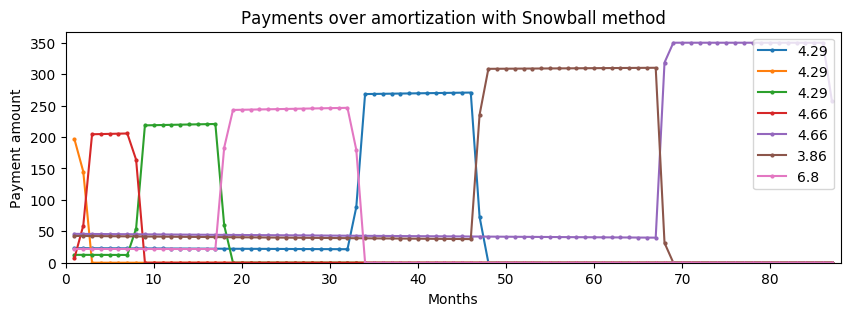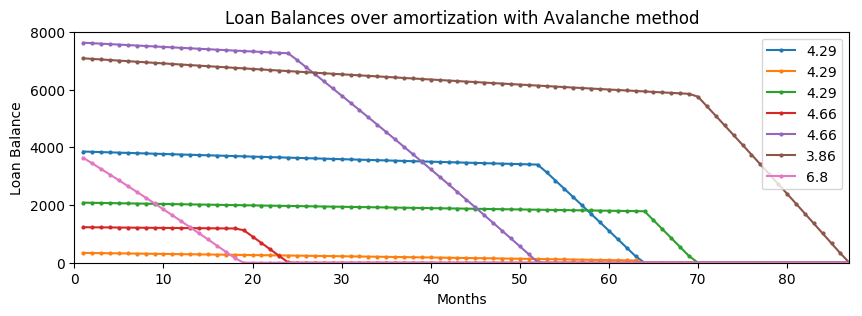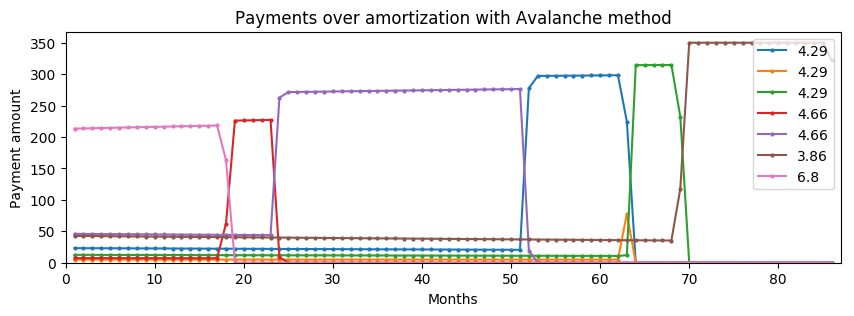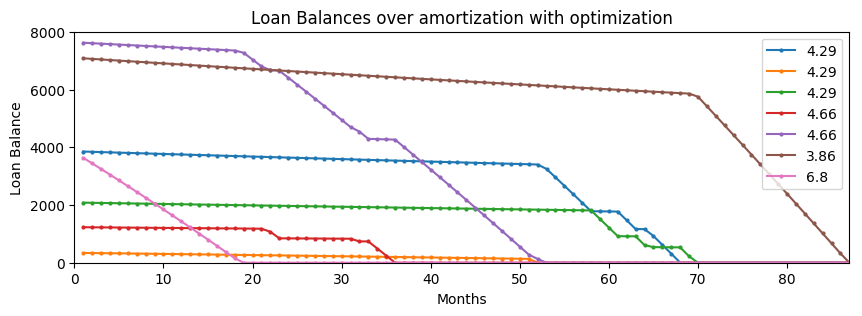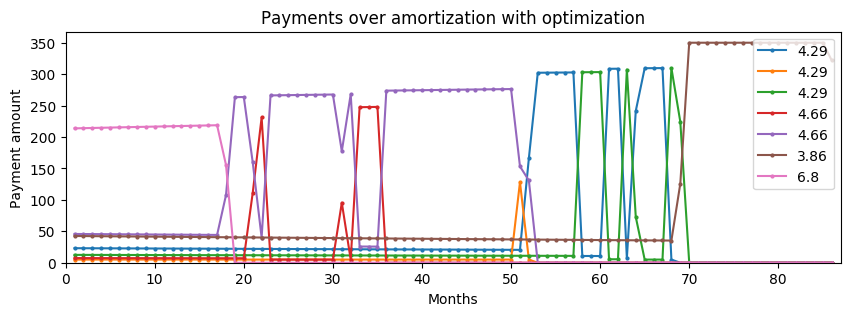The balance plots are satisfying to view as all the balances steadily go to zero. Looking at the payment plots, we notice that they are all over the place! In all three cases, the payments change nearly every month since the minimum payments are changing. Even in the seemingly flat sections, the payments are steadily changing. Most graduates do not want to update their payment amounts every month, since loan services typically allow automated payments and it is tedious to constantly change them, as well as somewhat demoralizing to look at the remaining loan balance every month. ### 4.D. Sparsity Analysis¶ We can address this problem by adding another objective: minimizing the difference between loan payments from month to month. Using an L1 norm on these differences will encourage sparsity in the solution, which is what we want! This secondary objective turns this into a trade-off problem. We can examine the effects of enforcing sparsity on our total payment, and look for a balance between sparse payments and minimal total payment. In : # plot tradeoff curve using PyPlot figure(figsize=(8,4)) plot(tradeoff_diffsums, tradeoff_totals, "-s",markersize=7,label="Optimized Cost") xpoints = [minimum(tradeoff_diffsums),maximum(tradeoff_diffsums)] plot(xpoints, [snowball_total, snowball_total], label="Snowball Cost") plot(xpoints, [avalanche_total, avalanche_total], label="Avalanche Cost") axis([xpoints,xpoints,.98*minimum(tradeoff_totals),1.02*maximum(tradeoff_totals)]); xlabel("Sum of Differences") ylabel("Total Cost") title("Total Cost vs Differences in payments") legend(loc="upper right",fancybox="true") ; # plot λ curve using PyPlot figure(figsize=(8,4)) semilogx(lams, tradeoff_totals, "-o",label="Optimized Cost") plot([lams,lams[end]], [snowball_total, snowball_total],label="Snowball Cost") plot([lams,lams[end]], [avalanche_total, avalanche_total],label="Avalanche Cost") axis([lams,lams[end],.98*minimum(tradeoff_totals),1.02*maximum(tradeoff_totals)]); xlabel("λ") ylabel("Total Cost") title("Total Cost vs Tradeoff Parameter") legend(loc="upper left",fancybox="true") ;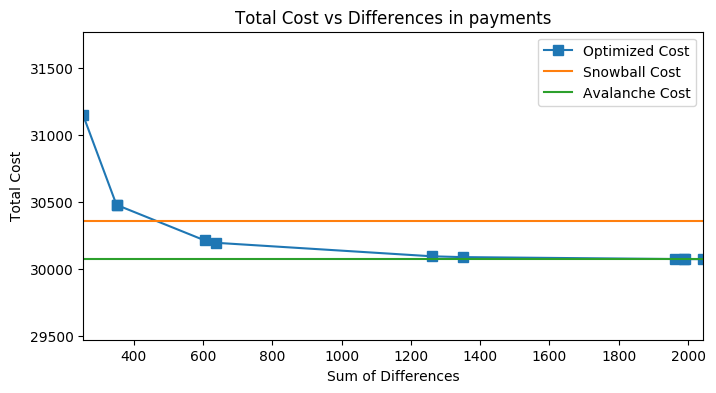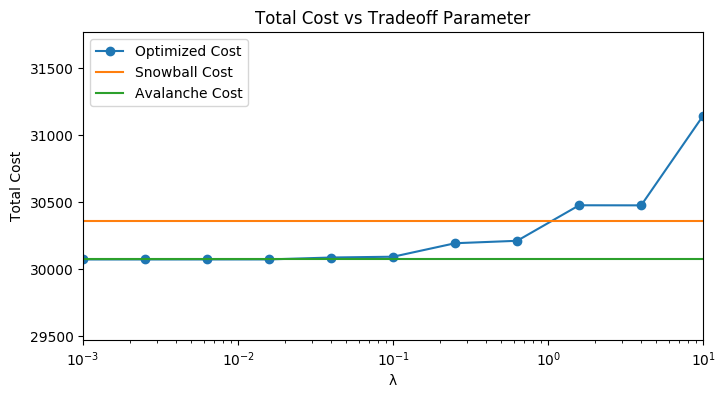The horizontal lines plotted indicate the costs of the snowball and avalanche methods. Ideally, we would be able to do better than both methods while still enforcing some sparsity into the optimization. However, considering the non-sparse solution just barely beats the avalanche method, we will have to settle for something in between. It looks like if we choose$\lambda = 10^{-1}$, we can do just about the same as the avalanche method but still have a substantial amount of sparsity. Here are the results of that choice. In : ## Total Cost and interest paid println(@sprintf("With λ chosen as %.1e",λopt)) println(@sprintf("Loan total is \$%.2f",total_principal))
println(@sprintf("Total payoff cost will be \$%.2f", totalλopt)) println(@sprintf("Interest paid will be \$%.2f",totalλopt-total_principal))
println(@sprintf("The loans will be paid off in %d months", num_monthsλopt))

println(@sprintf("Optimizing saves \$%.2f over snowball approach", snowball_total-totalλopt)) println(@sprintf("Optimizing saves \$%.2f over avalanche approach", avalanche_total-totalλopt))
println(@sprintf("Optimizing with sparsity costs \$%.2f over non-sparse approach", -(orig_total-totalλopt)))  With λ chosen as 1.0e-01 Loan total is$25850.08
Total payoff cost will be $30092.37 Interest paid will be$4242.29
The loans will be paid off in 87 months
Optimizing saves $265.42 over snowball approach Optimizing saves$-20.13 over avalanche approach
Optimizing with sparsity costs $20.48 over non-sparse approach  So, enforcing sparsity costs us about$20 over the course of the amortization compared to the optimized, non-sparse solution or the avalanche method. However, the sparse solution still beats the snowball method by a good margin.

What about the sparseness? We enforced sparsity so that the montly payments would have to be changed less often. How good is this solution for that purpose? Let's examine the payment plot.

In :
# plot payments with chosen λ
using PyPlot
figure(figsize=(8,3))
for ll in 1:size(paymentsλopt)
plot(collect(1:num_monthsλopt), paymentsλopt[:,ll], "-o",markersize=2,)
end
axis([0,num_monthsλopt,0,1.1*maximum(paymentsλopt)]);
xlabel("Months")
ylabel("Payment amount")
title(@sprintf("Payments over amortization with chosen λ = %.1e",λopt))
;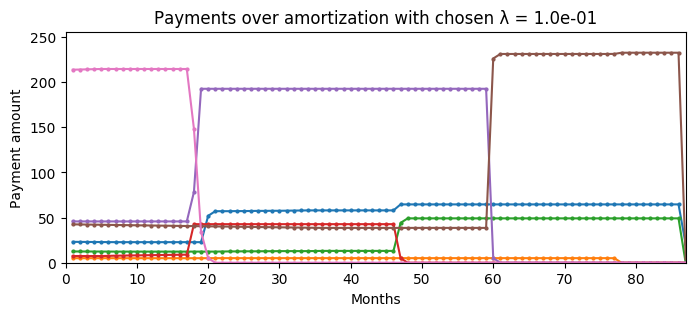This looks great. The payments over time are nice and flat for long stretches. It's easy to calculate the precise number of times that the payments change. To be realistic, we can ignore times that the payments change less than 50 cents. The graduate student probably won't take the time to make that adjustment, and there won't be much of a change in the amortization.

In :
payment_diffs = zeros(size(paymentsλopt))
for rr in 1:size(paymentsλopt)-1
payment_diffs[rr,:] = paymentsλopt[rr+1,:]-paymentsλopt[rr,:]
end
sum(sum(abs.(payment_diffs),2).>0.5)

Out:
10

Only 10 payment changes over the course of 86 months! That seems very reasonable, and I'm sure the graduate will appreciate spending less time on their loan servicer's website instead of logging in every month to look at the outstanding balance.

The L1 norm had the desired effect on the optimization. By added that value to the objective, the solver found a solution that has only sparse changes in payments from month to month. We can visualize this with a plot of the sum of changes on a monthly basis.

In :
figure(figsize=(8,3))
plot(sum(abs.(payment_diffs),2),"r-o")
title("Total Change in Payments in Each Month of Amortization")
xlabel("Month")
ylabel("Sum of changes (\$)") ;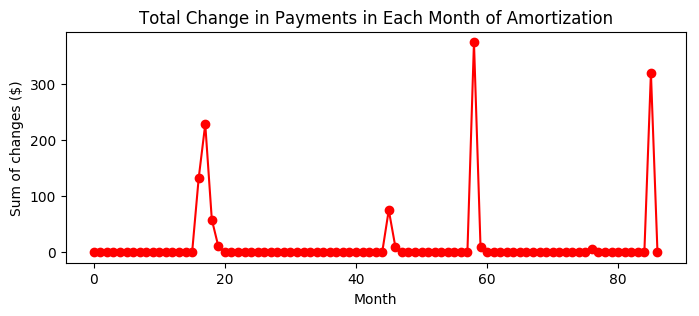As this plot shows, the solver found a very sparse solution. While still having a fairly optimal approach to paying off loans, it found a way to group the changes into clumps while most months, there's no change at all. There are 4 main peaks in this plot and one tiny one. During those times, the solution transitions from completing the payoff of some loans and switching focus to others. ## 5. Conclusion¶ First, let's review the problems for which we wanted solutions. 1. Student loans come in bunches, not just a single big loan. This makes payoff complicated. What is an optimal way to pay off multiple loans at once? 2. There are two dominant strategies outlined on the web for multiple loan payoff: the snowball and avalanche methods. How do these method compare, and is there a better strategy? 3. A graduate might be working on a budget and trying to decide how much to contribute to paying off loans each month. What are the consequences of various amounts? 4. We found that the optimal solution, as well as the snowball and avalanche methods, had constantly changing payments. Is there a way to have more stable payments? 5. After reforming the problem to have more stable payments, it became a tradeoff. What are the consequences of the tradeoff, and what does one of the solutions look like? So, what have we learned? 1. We were able to model multiple-loan amortization with a mixed-integer linear program. The model is fairly simple, with the exception of properly accounting for the minimum payments allowed, which was important. This model was solved to find the optimal solution for the given loan parameters. 2. We simulated payoff of loans with the two proposed strategies as well. We found that the snowball method is clearly less optimal than other methods, although there's other supporting reasons for it. We found the avalanche method is surprisingly good- our optimal solution only beats it by \$.40! (for the given loan parameters)

3. We did a quick examination of varying the monthly payment towards loans. The law of diminishing returns is in effect. A graduate can save a lot of money and time paying off their loans if they had a small monthly payment in mind and decide to add a little more. However, if they have a significantly large monthly payment already, they won't experience much difference with paying a little more. A graduate could put their own personal loan balances in and make use of this analysis when planning their budget.

4. After seeing how the payments are constantly changing with the optimal solution, we reformatted the model to become a tradeoff between minimizing the total amount paid and minimizing the change in payments. We used an L1 norm in the model to encourage sparsity in the solution, so updates would only have to be made to the monthly payments occasionally.

5. Once the new model was made, we solved it for multiple values of the tradeoff parameters, $\lambda$. It turns out that it quickly adds to the total cost of payoff to find a highly sparse solution. However, we could add some sparsity while still keeping the overall cost close to the original optimal amount. The resulting solution was quite pleasing. By only sacrificing \\$20 over the course of amortization, the payments only need to be adjusted 10 times.

A graduate can get a lot of utility out of this analysis, including finding optimal payments, deciding on a budget, and finding ways to spend less time adjusting monthly payments.

Some other considerations that were not dealt with in this project:

1. We only examined a single set of loan parameters in depth. While I checked multiple different parameters to ensure that there were no qualitative surpirses, it would be useful to see an in-depth analysis of the interplay between the different changing parameters.

2. A maximum monthly payment budget was assumed constant throughout amortization. However, any recent college graduate can tell you that budgets are never that constant. There are both random perturbances, such as an expensive month or an influx of cash, and there are steady changes, like raises or different salaries over time. It would be useful to analyze how these effects change the optimal strategy, and perhaps explore how to find a solution that is more robust to random changes.

3. This project focused on student loans, but there are other scenarious in which an individual could be paying multiple loans at once. For exampe, such as credit card debt, car loan, and mortgage. These loans could start at different time points so the model would have to be adjusted to account for that.

In conclusion, this work can hopefully provide some guidance and peace of mind for a graduate facing the daunting task of paying off student debt. While paying off a mountain of debt in the form of multiple loans is still intimidating, at least one can know they are doing it optimally.